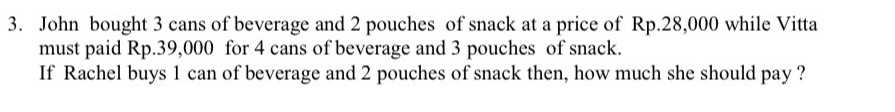### ¿Todavía tienes preguntas de matemáticas?

Pregunte a nuestros tutores expertos
Algebra
Pregunta3. John bought $$3$$ cans of beverage and $$2$$ pouches of snack at a price of $$R p .28,000$$ while Vitta must paid Rp. $$39,000$$ for $$4$$ cans of beverage and $$3$$ pouches of snack. If Rachel buys $$1$$ can of beverage and $$2$$ pouches of snack then, how much she should pay?